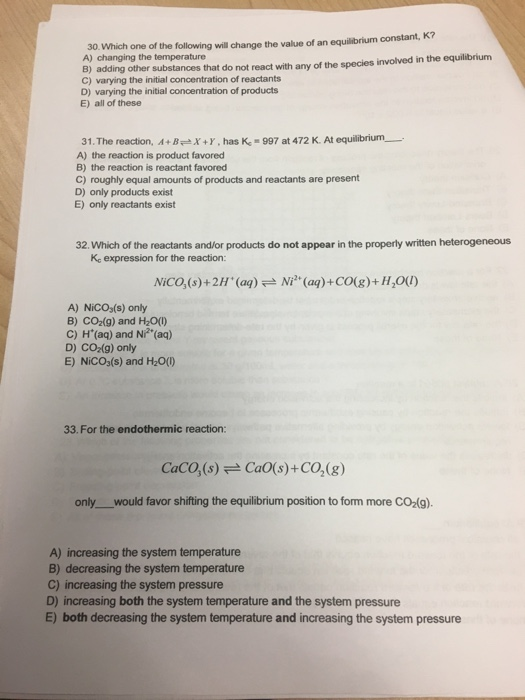# Question & Answer: Which one of the following will change the value of an equilibrium constant, K? A) changing…..Which one of the following will change the value of an equilibrium constant, K? A) changing the temperature B) adding other substances that do not react with any of the species involved in the equilibrium C) varying the initial concentration of reactants D) varying the initial concentration of products E) all of these The reaction, A + B X + Y, has K_c = 997 at 472 K. At equilibrium _____. A) the reaction is product favored B) the reaction is reactant favored C) roughly equal amounts of products and reactants are present D) only products exist E) only reactants exist Which of the reactants and/or products do not appear in the properly written heterogeneous K_c expression for the reaction: NiCO_3 (s) + 2H^+ (aq) Ni^2+ (aq) + CO (g) + H_2O (l) A) NiCO_3(s) only B) CO_2(g) and H_2O(l) C) H^+(aq) and Ni^2+(aq) D) CO_2(g) only E) NiCO_3(s) and H_2O(l) For the endothermic reaction: CaCO_3(s) CaO(s) + CO_2(g) only _____ would favor shifting the equilibrium position to form more CO_2(g) A) increasing the system temperature B) decreasing the system temperature C) increasing the system pressure D) increasing both the system temperature and the system pressure E) both decreasing the system temperature and increasing the system pressure

1.Equilibrun constant is function of temperature only and hence change in temperature changes the equilibrium constant. If concentration changes, new equilibrium is established without distrubing the equilibrium constant. ( A is correct)

2. Gibbs free energy (deltaG)= -RT lnK, -ve value of deltaG favors the forward reaction. for that K has to be >1. in the present case K is >1, deltaG is -ve and the reaction is product favored.

Don't use plagiarized sources. Get Your Custom Essay on
Question & Answer: Which one of the following will change the value of an equilibrium constant, K? A) changing…..
GET AN ESSAY WRITTEN FOR YOU FROM AS LOW AS \$13/PAGE

3. when writing the expression for equilibrium constant, the pure substance in liquid form and the solid will not be seen in the expression becasue their activity is 1. Hence in the present case, NICO3(s) and H2O(l) will not appear in the equilibrium constant equation.( E is correct)

4 . for the reaction, CaCO3(s)——->CaO(s)+CO2(g)

K= pCO2, p = pressure of CO2. Higher the pressure of CO2, higher the value of CO2 and hence higher the reaction to the right. The reaction is endothermic so increase in temperature as per Lechatlier principle favors the endothermic direction. So high pressure and high temperature favors the formation of CO2. ( E is correct)